• 基于梯度下降的参数辨识代码，分析了定常和时变系统在不同学习率调整规则下的辨识结果。在MATLAB 的AppDesigner设计工具上制作了参数辨识面板。
• 这是可执行的自适应遗忘因子参数辨识代码，希望能够帮到你
• 六维力传感器参数辨识方法+matlab代码实现一、理论二、matlab三、验证 一、理论 此参数辨识方法源自于中国科学院沈阳自动化研究所的博士学位论文《基于力控制的工业机器人精密装配研究》，提出感谢！ 算法如下： ...
六维力传感器参数辨识方法+matlab代码实现一、理论二、matlab三、验证
一、理论
此参数辨识方法源自于中国科学院沈阳自动化研究所的博士学位论文《基于力控制的工业机器人精密装配研究》，提出感谢！
算法如下：二、matlab
资源地址
三、验证
参数：效果：展开全文• 六关节机械臂动力学参数辨识 导语：两周的动力学参数辨识，使我学到很多，但遇到的问题更多，在网上有很多六关节动力学参数辨识的资料，但大家都是理论推导六关节，仿真时却是对二三关节来进行辨识，感觉有点...
导语：两周的动力学参数辨识，使我学到很多，但遇到的问题更多，在网上有很多六关节动力学参数辨识的资料，但大家对于最小惯性参数集的推导都不详细，不能得到最小惯性参数集的系数和对应的回归矩阵，很多东西都是直接给出来了，这期间我自己搭建模型，写代码，目前成功辨识出来第六关节的惯性参数，但在辨识第五关节的惯性参数时遇到一点问题，正在解决中，下篇推出全部关节的辨识
动力学参数辨识过程
熟悉动力学参数辨识的人都明白辨识过程，过程如下，不再赘述
辨识过程：1.建立机械臂动力学模型
2.机器人动力学模型线性化并整理出最小参数集(难点)
3.激励轨迹的设计及其优化
4.动力学模型的参数辨识及其验证
第一步 建立机械臂动力学模型
动力学方程
$\boldsymbol{M}\left( \boldsymbol{q} \right) \boldsymbol{\ddot{q}}+\boldsymbol{c}\left( \boldsymbol{q},\boldsymbol{\dot{q}} \right) +\boldsymbol{G}\left( \boldsymbol{q} \right) =\boldsymbol{\tau }$
三种建模方法，牛顿-欧拉动力学建模方法应用最广泛，便于编程实现，网上也有资源，不多说。
第二步 机器人动力学模型线性化并整理出最小参数集
将第一步的动力学模型线性化如下：
$\boldsymbol{\varPhi }\left( \boldsymbol{q},\boldsymbol{\dot{q}} \right) \cdot \boldsymbol{\theta }\,\,=\,\,\boldsymbol{\tau }$
$\boldsymbol{\theta }\,\,是\text{要辨识的惯性参数，}\boldsymbol{\varPhi }\text{矩阵}是\text{参数矩阵，}是\boldsymbol{q}\text{和}\boldsymbol{\dot{q}}\text{的方程}$
我参考的书籍是：机器人动力学与控制第二章第五节.机器人的最小惯性参数及其应用
这本书可以说是动力学参数辨识的鼻祖，是最原始的讲解，对于何种机械臂，最小惯性参数应该是多少，这本书里面均有着详细的讲解，网上也有资源，可以免费下载。
对于6R机械臂，输入六个关节的DH参数，输出最小参数集为36个，参数如下注：这里暂未考虑摩擦系数，只有36个参数，如果考虑到摩擦，则有36+12=48个参数。
第三步 激励轨迹的设计及其优化
目前工业机器人动力学参数辨识大都采用傅里叶级数型的轨迹，这里我选择
5*cos(t) + 10*cos(2*t)

作为激励轨迹，轨迹的优化暂不考虑。
第四步 动力学模型的参数辨识
在进行这步之前，需搭建好你的机械臂控制器，机械臂动力学模型，辨识模块(这里采用RLS辨识)

机械臂控制器模块：根据动力学模型建立的滑模控制器，能够跟踪理想的关节角度，关节角速度。
动力学模型：牛顿欧拉动力学方程或者凯恩方法建立。
辨识模块：递推最小二乘法辨识机械臂动力学模型中各关节的惯性参数真实值为：我使第五，六关节的关节角为激励轨迹5cos(t) + 10cos(2*t)，其余四个关节角为0，启动仿真，仿真结果如下
六个关节角的运动角度曲线：可以看到第五六关节与期望轨迹吻合，且其余四个关节角均保持在1e-11次方左右，可认为是0，达到了角度控制的效果，控制器设计良好。
第六关节惯性参数辨识结果：在参数辨识图中，第一个小图辨识的值为L_6xx-L_6yy的组合值，第二个小图辨识的值为L_6xy，第三个小图辨识的值为L_6xz，第四个小图辨识的值为L_6yz，将辨识结果与真实值比较可得，辨识效果很好，均得到准确的辨识。第一个小图辨识的值为L_6zz，第二个小图辨识的值为l_6x，第三个小图辨识的值为l_6y，比较可得，辨识准确，第六关节惯性参数得到了准确的辨识。
遇到问题：在辨识出第六关节的惯性参数后，应该将辨识值作为已知值代入到第五关节的辨识程序中来辨识第五关节的惯性参数，但我遇到的问题是线性化后的第五关节的力矩和线性化之前的牛顿欧拉动力学的力矩不相等，导致第五关节惯性参数不能正确辨识，现正在调试中，期待不久能够解决。未完待续…


展开全文matlab
• 前言最近看了几篇关于传染病模型...有了动力学模型也就必然会有模型参数辨识，而模型参数辨识往往可以被建模为一个优化问题，而优化问题的求解也是我的老本行运筹学了。在此声明，本文的模型还是比较toy的，能有...
前言最近看了几篇关于传染病模型的科普文章觉得很有趣，于是自己动手撸了一遍。虽然貌似传染病模型和运筹学和控制论好像没有关系，实际上传染病模型很多都是动力学模型（常微分方程），这些模型我们在Control theory里边并不陌生哈。有了动力学模型也就必然会有模型参数的辨识，而模型参数的辨识往往可以被建模为一个优化问题，而优化问题的求解也是我的老本行运筹学了。在此声明，本文的模型还是比较toy的，能有多少准确性很难保证，不过我觉得是一个很好的练手的project，毕竟作为一个科研工作者我们以我们的方式来做点什么。1 SIR模型简介SIR模型是常见的一种描述传染病传播的数学模型，其基本假设是将人群分为以下三类：1 易感人群（Susceptible）：指未得病者，但缺乏免疫能力，与感病者接触后容易受到感染。2 感染人群（Infective）：指染上传染病的人，他可以传播给易感人群。3 移除人群（Removed）：被移出系统的人。因病愈（具有免疫力）或死亡的人。这部分人不再参与感染和被感染过程。如下图所示，在SIR 模型中以上三类人群之间存在两个转换的关系：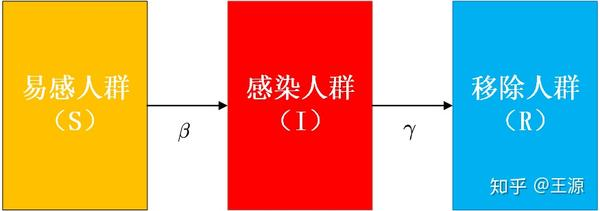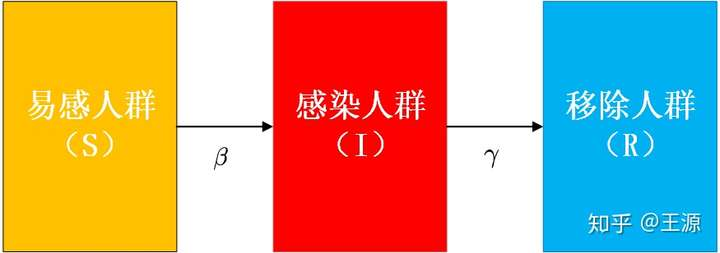易感人群与感染人员接触时被传染，传染率为。传染率反映了疾病传播的强度，传染率越大则易感人群和感染人员接触后被传染的可能性越大。感染人群以固定平均速率  恢复或死亡。恢复率，取决于感染的平均持续时间 。简化起见分别用三类人群的首字母表示三类人群的数量，表示人工总数。那么三类人群数量随时间的动态变化的规则可用以下常微分方程组表示：（1）（2）（3）2 采用优化算法对传染率进行参数辨识通过上面的介绍我们知道SIR模型实际上是采用动力学模型（三个常微分方程）对三类人群随时间变化的过程进行建模，采用传染率和恢复率来量化描述疾病传染和疾病被治愈等行为。很重要的一点是只有获得准确的动力学模型参数才有可能建立一个相对精确的模型。那么要采用SIR模型对武汉新型肺炎传播进行建模其主要问题就是确定出以下参数1 传染率和 恢复率2 易感人群（Susceptible）初值，感染人群（Infective）初值，移除人群（Removed）初值由于第一例病例是在12月8日被确诊，因此选择初始时间在12月8日。感染人群初值为1，易感人群初值为，移除人群初值为0，其中为武汉市总人口数。为恢复率，因为新型冠状病毒肺炎的恢复期大约是14天，因此取。因此下面我们主要是围绕如何辨识出准确的传染率为了方便进行参数辨识，我们对上述SIR模型进行一个简化，我们认为在疾病传播的早期有(传播早期患病人数较少，所以可以近似认为所有人都是易感人群)，将这个条件带入到式（2）中可得（4）易知该微分方程的通解为：(5)由可得，带入式（5）中可得(6)由此可以构建如下参数辨识问题：决策变量：传染率目标函数：（7）其中为实际的患病人数(从实际数据来)，为时间集合，以天为单位。通过求解上述优化问题即可得到武汉新型肺炎的传染率，易知该优化问题是一个非线性非凸的优化问题。3 参数辨识所需数据获得与选取从上面的参数辨识问题可以看出，我们需要武汉市新型肺炎患病的历史数据（主要是每天的患病人数）。我们从github上下载了全国主要城市1月20日至2月1日患病人数的数据(数据来源：839Studio/Novel-Coronavirus-Updates)，其数据格式如下：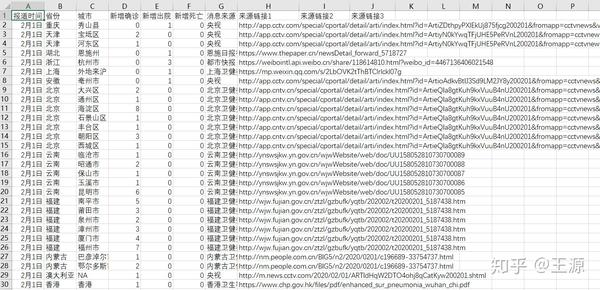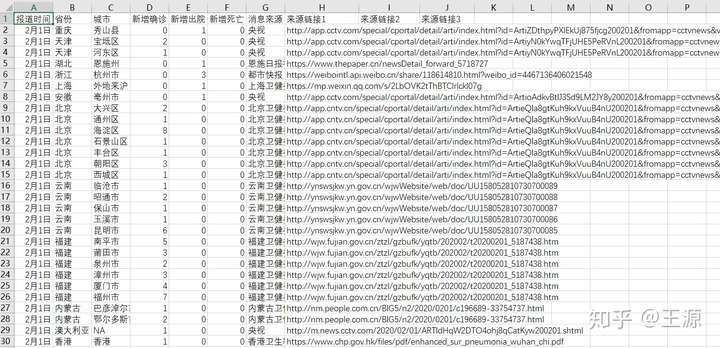我们从中提取武汉市1月18日至1月22日的数据来进行参数辨识为何选取18日至22日的数据？因为武汉封城是在23日，在封城之前疾病的传播受到人为因素影响较小，所以采用封城前的数据来做参数辨识。同时由于18日之前武汉患病数据可能并不真实（有瞒报情况），所以18日之前的数据也不采用。通过数据预处理后，得到用来辨识的数据格式如下所示：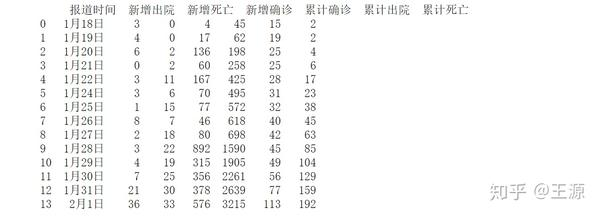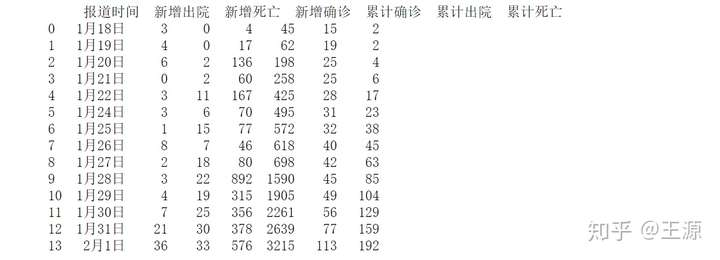4 Python代码实现数据处理要用到Numpy和Pandas，解微分方程和求解辨识问题需要Scipy，画图需要Matplotlibimport numpy as np
import pandas as pd
import math
import datetime
from scipy.integrate import odeint
from scipy.optimize import minimize
import matplotlib.pyplot as plt主干代码分为三大块，1是数据预处理部分，主要功能是从原始数据中提取出我们辨识问题所需的数据，2是辨识问题部分，主要功能是求解优化问题，得到准确的传染率，3是SIR模型，主要是动力学模型的求解。1 数据预处理部分，原始数据存储在Updates_NC.csv文件中，主要是提取出武汉市的数据，然后计算出每天累计患病人数，最后提取1月18日之后的数据。Updates_NC = pd.read_csv('Updates_NC.csv')
class preProcess():
def __init__(self):
wuHanInfection = self.wuHan.groupby('报道时间')['新增确诊'].sum()
wuHanRecovered = self.wuHan.groupby('报道时间')['新增出院'].sum()
self.wuHan = {'报道时间':wuHanInfection.index, '新增确诊':wuHanInfection.values, '新增出院': wuHanRecovered.values, '新增死亡':wuHanDead.values}
self.wuHan = pd.DataFrame(self.wuHan, index = [i for i in range(wuHanInfection.shape)])
def getTotal(self):
wuHanTotalInfection = [self.wuHan.loc[0:i,'新增确诊'].sum() for i in range(self.wuHan.shape)]
wuHanTotalRecovered = [self.wuHan.loc[0:i,'新增出院'].sum() for i in range(self.wuHan.shape)]
wuHanTotalDead = [self.wuHan.loc[0:i,'新增死亡'].sum() for i in range(self.wuHan.shape)]
self.wuHan = self.wuHan.join(pd.DataFrame([wuHanTotalInfection,wuHanTotalRecovered ,wuHanTotalDead], index = ['累计确诊', '累计出院','累计死亡']).T)
print(self.wuHan)

def removeNoisyData(self):
self.wuHan = self.wuHan[self.wuHan['报道时间'] &gt;= '1月18日']
self.wuHan.index = [i for i in range(self.wuHan.shape)]
print(self.wuHan)

def report(self):
plt.plot(self.wuHan.index, self.wuHan['累计确诊'])
plt.xlabel('Day')
plt.ylabel('Number of people(Wu Han)')
plt.show()</code></pre></div><p>2 定义出参数辨识问题，主要是定义出目标函数（costfunction）即可调用Scipy来帮助我们求解优化问题。实际上在代码中我们在求解辨识问题的时候，将传染率拆成了2项相乘的形式 <img src="https://www.zhihu.com/equation?tex=%5Cbeta%3DnContact+%5Ctimes+infectionProb+" alt="[公式]" eeimg="1" data-formula="\beta=nContact \times infectionProb "> ，其中 <img src="https://www.zhihu.com/equation?tex=nContact" alt="[公式]" eeimg="1" data-formula="nContact"> 为感染人员每天接触的正常人的数量（假设为5人）， <img src="https://www.zhihu.com/equation?tex=infectionProb" alt="[公式]" eeimg="1" data-formula="infectionProb"> 为感染概率</p><div class="highlight"><pre><code class="language-text">class estimationInfectionProb():
def __init__(self, estUsedTimeIndexBox, nContact, gamma):
self.timeRange = np.array([i for i in range(estUsedTimeIndexBox,estUsedTimeIndexBox + 1)])
self.nContact, self.gamma = nContact, gamma
self.dataStartTimeStep = 41

def setInitSolution(self, x0):
self.x0 = 0.04

def costFunction(self, infectionProb):
#print(infectionData.wuHan.loc[self.timeRange - self.dataStartTimeStep,'累计确诊'])
#print(np.exp((infectionProb * self.nContact - self.gamma) * self.timeRange))
res = np.array(np.exp((infectionProb * self.nContact - self.gamma) * self.timeRange) - \
infectionData.wuHan.loc[self.timeRange - self.dataStartTimeStep,'累计确诊'])
return (res**2).sum() / self.timeRange.size

def optimize(self):
self.solution = minimize(self.costFunction, self.x0, method='nelder-mead', options={'xtol': 1e-8, 'disp': True})
print('infection probaility: ', self.solution.x)
return self.getSolution()

def getSolution(self):
return self.solution.x

def getBasicReproductionNumber(self):
self.basicReproductionNumber = self.nContact * self.solution.x / (self.gamma)
print("basic reproduction number:", self.basicReproductionNumber)
return self.basicReproductionNumber</code></pre></div><p>定义出SIR 模型的类，代码写得比较直观和简单，此处就不多解释了，相信很容易看懂。主要是用scipy来求解常微分方程，即式（1-3）。需要注意的是此处的传染率 <img src="https://www.zhihu.com/equation?tex=%5Cbeta" alt="[公式]" eeimg="1" data-formula="\beta"> 要用上面参数辨识得到的值。</p><div class="highlight"><pre><code class="language-qvto">class wuHanSIRModel():
def __init__(self, N, beta, gamma):
self.beta, self.gamma, self.N = beta, gamma, N
self.t = np.linspace(0, 360, 361)
self.setInitCondition()

def odeModel(self, population, t):
diff = np.zeros(3)
s,i,r = population
diff = - self.beta * s * i / self.N
diff = self.beta * s * i / self.N - self.gamma * i
diff = self.gamma * i
return diff

def setInitCondition(self):
self.populationInit = [self.N - 1, 1, 0]

def solve(self):
self.solution = odeint(self.odeModel,self.populationInit,self.t)

def report(self):
#plt.plot(self.solution[:,0],color = 'darkblue',label = 'Susceptible',marker = '.')
plt.plot(self.solution[:,1],color = 'orange',label = 'Infection',marker = '.')
plt.plot(self.solution[:,2],color = 'green',label = 'Recovery',marker = '.')
plt.title('SIR Model' + ' infectionProb = '+ str(infectionProb))
plt.legend()
plt.xlabel('Day')
plt.ylabel('Number of people')
plt.show()

最近在家上github很慢，我就把全套代码放到网盘上吧。网盘链接如下： https://pan.baidu.com/s/1X4oiPhU5d8CQX01DOg6zPA 提取码: abqa5 结果分析1 武汉市患病人数分析如下图所示是武汉市患病人数数据，用红色框圈出的是累计患病人数，可以很明显地看到从1月11日至1月18日的累计患病人数基本保持不变，这部分数据的可信度还需要进一步考量。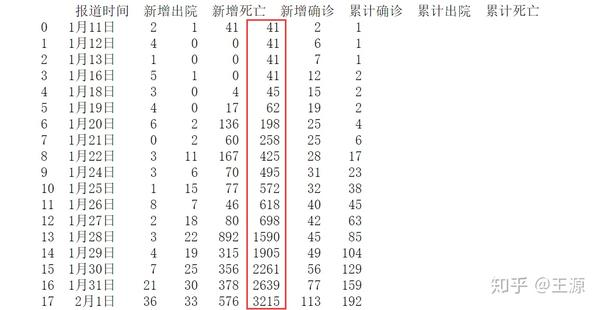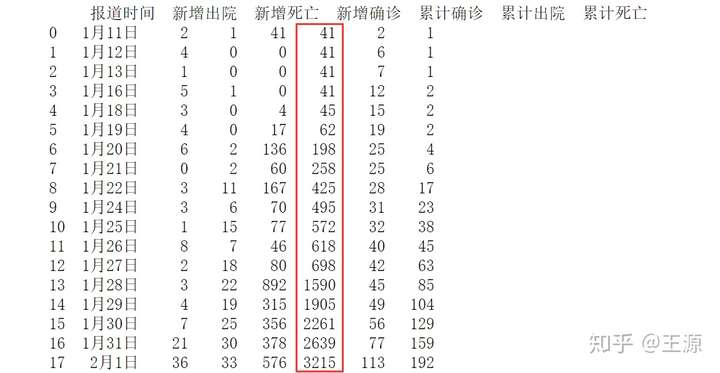2 传染率辨识结果分析我们得到的辨识结果为 infection probaility:   0.03926041，传染率= 0.19630同时可以采用如下公式估算出 武汉新型肺炎病毒基本传染数（basic reproduction number）基本传染数是指在没有外力介入，同时所有人都没有免疫力的情况下，一个感染某种传染病的人，会把疾病传染给其他多少个人的平均数，基本传染数是衡量疾病传染性的一个重要指标。若则传染病将会逐渐消失，若传染病会以指数方式散布，成为流行病。非典的基本传染数约为0.85-3，埃博拉基本传染数约1.5-2.5。3 用SIR模型预测武汉市患病人数定义nContact为 每个患病人员每天会接触nContact个正常人，nContact越大表明隔离和管制措施越弱，同时接触后正常人的患病概率为0.03926的情况下SIR模型结果如下即若不采取任何管制和防控措施的条件下，假设nContact=5，武汉市患病人数随时间变化的曲线如下所示：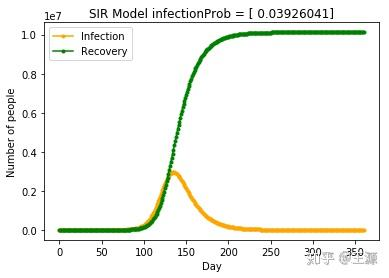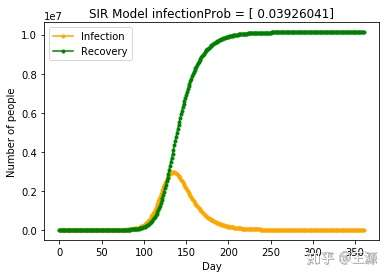当nContact=4，武汉市患病人数随时间变化的曲线如下所示：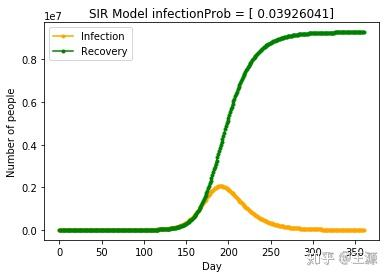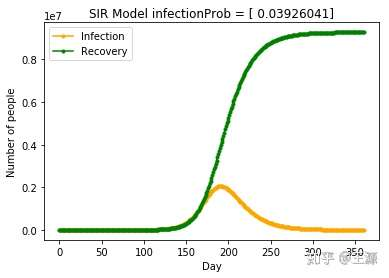当nContact=3，武汉市患病人数随时间变化的曲线如下所示：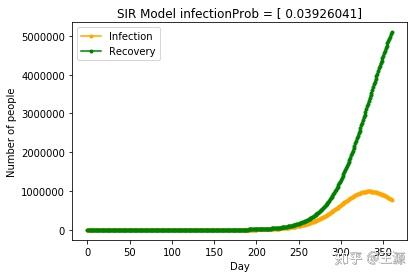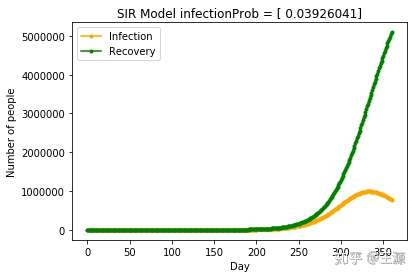当nContact=2 (表明已经采用了比较严厉的隔离和管制措施)，武汉市患病人数随时间变化的曲线如下所示：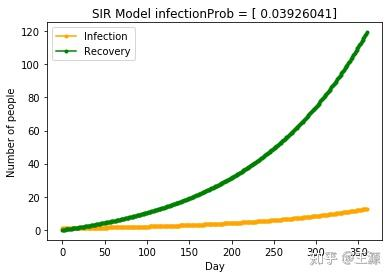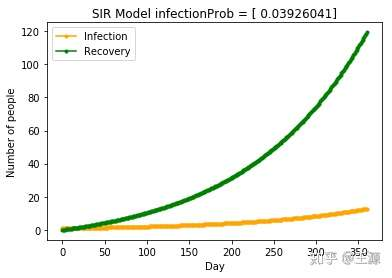从上面的结果可以非常清晰得看出随着nContact的减少，患病人数急剧减少，当nContact=2时 患病人数甚至不超过100人，可见采取严厉的管制和防控隔离措施对武汉市新型冠状病毒患病人数的减少有着非常明显的效果。6 总结1 采用动力学模型对疾病传播建模并不难，难点在于如何利用真实数据辨识动力学模型中的参数。2 对真实数据的收集，预处理等工作看起来不起眼，实际上在我们的工作中是一个非常重要的部分。3 SIR模型还是比较粗糙的一个模型，主要是没有考虑到潜伏期，那么SEIR模型会更加精确一些，后期有兴趣可以再做一做。4 还可以考虑两阶段的模型，例如武汉市在1月23日采取了封城措施，那么可以将封城前后分别进行建模，构成一个两阶段模型会更加准确一些。参考文献：小烎模：武汉肺炎传播的多种数学模型​zhuanlan.zhihu.com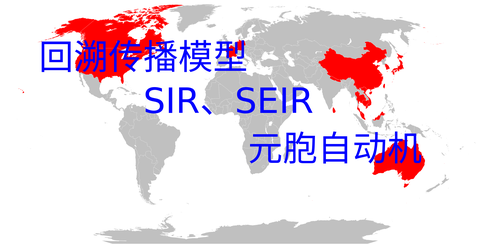张戎：传染病的数学模型​zhuanlan.zhihu.com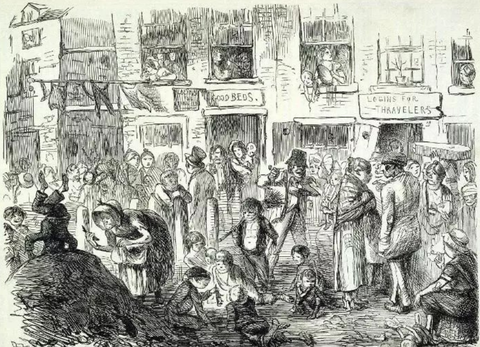【1】新型冠状病毒的疫情评估与预测报告，北京航空航天大学计算机学院智慧城市（BIGSCity）课题组和经管学院数据智能（DIG）课题组


展开全文• 结果表明:该改进型粒子群算法辨识GMA输出位移模型参数有效性高,参数辨识代码运行时间缩短至210s,适应度函数值最小达到0. 165 7,由此建立的磁滞非线性模型的计算精度可精确到0. 001μm,且通过多次比较发现该位移输出...
• IMU噪声参数辨识-艾伦方差前言推导过程1. 量化噪声（Quantization Noise，QN）2．角度随机游走（Angular Random Walk，ARW）3．零偏不稳定性噪声（Bias Instability，BI）4．角速率随机游走（Rate Random Walk，RRW...
IMU噪声参数辨识-艾伦方差前言推导过程1. 量化噪声（Quantization Noise，QN）2．角度随机游走（Angular Random Walk，ARW）3．零偏不稳定性噪声（Bias Instability，BI）4．角速率随机游走（Rate Random Walk，RRW）5．速率斜坡（Rate Ramp，RR）5种艾伦方差汇总介绍与单位转换艾伦方差辨识参数的误差问题艾伦方差曲线的绘制方法艾伦方差相关代码艾伦方差应用实例1.ADIS164651.ADIS164703.某型号光纤惯导10s平滑计算零偏不稳定性参考文献
前言
在统计学中描述随机变量的两个经典参数是均值和方差，早期在定量表征原子钟的频率稳定度时采用的就是经典方差方法。1996年，学者D.W.Allan在分析铯原子钟频标的频率稳定度时发现经典方差随着时间的增长而发散，为了解决该问题，提出了一种新的评定方法，后来称为艾伦方差。由于惯性器件也具有振荡器的特征，Allan方差分析也被广泛应用于惯性器件的随机误差建模，IEEE标准中就将Allan方差方法引入到了激光陀螺的建模分析。
Allan方差的物理意义以及应用本质来源于它与功率谱之间的关系，本文首先从功率谱入手推导艾伦方差，然后教大家如何从艾伦方差log曲线中辨识IMU的各种噪声，最后会给出Allan方差的实例应用，包括光纤惯导和Mems惯导，并且给出了和10s平滑方法的差异。
推导过程
因为内容较多，这里只会给出一个大概的推导流程，更多细节的推导可以参考严恭敏老师《惯性仪器测试与数据分析》一书。
从时域测量方面来分析，Allan方差定义如下:
$\sigma_{y}^{2}(\tau)=\frac{1}{2}<\left[y_{i+1}(\tau)-y_{i}(\tau)\right]^{2}>$
然后是艾伦方差和功率谱密度之间的关系，如下所示：
$\sigma_{y}^{2}(\tau)=2 \int_{-\infty}^{\infty} S_{y}(f) \frac{\sin ^{4}(\pi f \tau)}{(\pi f \tau)^{2}} \mathrm{d} f=4 \int_{0}^{\infty} S_{y}(f) \frac{\sin ^{4}(\pi f \tau)}{(\pi f \tau)^{2}} \mathrm{d} f$
上式中$S_{y}(f)$为功率谱密度。
从艾伦方差曲线中可以辨识出IMU的五种噪声，分别为：量化噪声、角度随机游走、零偏不稳定性噪声，角速率随机游走，速率斜坡，一般在IMU噪声辨识中用的比较多的是中间3种。
下面分别介绍5种噪声和Allan方差之间的关系。
1. 量化噪声（Quantization Noise，QN）
量化噪声产生的来源是传感器在经过A/D转换环节，即存在模拟量向数字量的转化的量化过程，然后这一过程是必然存在信息损失的，所以量化噪声代表了传感器检测的最小分辨率水平。
角速率的量化噪声功率谱为：
$S_{\Omega}(f)=\tau_{0} Q^{2}(2 \pi f)^{2}$
代入艾伦方差和功率谱密度关系公式中得到：
$\sigma_{Q N}^{2}(\tau)=4 \int_{0}^{\infty} \tau_{0} Q^{2}(2 \pi f)^{2} \frac{\sin ^{4}(\pi f \tau)}{(\pi f \tau)^{2}} \mathrm{d} f=\frac{16 \tau_{0} Q^{2}}{\pi \tau^{3}} \int_{0}^{\infty} \sin ^{4}(\pi f \tau) \mathrm{d}(\pi f \tau)$
上公式化简整理可得：
$\log _{10} \sigma_{Q N}(\tau)=\log _{10} \sqrt{3} Q-\log _{10} \tau$
由此可见，在Allan方差$\tau-\sigma_{Q N}$双对数图上，量化噪声对应的斜率为-1，它（或延长线）与$\tau=1$交点的纵坐标读数为$\sqrt{3} Q$，如下图所示。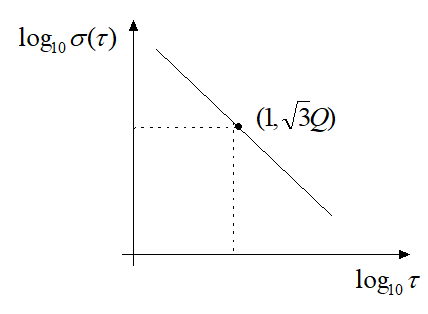这里需要注意一下，严格意义上应该称$\sigma(\tau)$为Allan标准差，而称$\sigma(\tau)^{2}$为Allan方差，但习惯上常常直接称$\sigma(\tau)$为Allan方差，以后在不引起歧义的情况下所说的Allan方差亦指$\sigma(\tau)$，在概念上不要造成混乱。
量化噪声具有很宽的带宽，属于高频噪声，在实际应用中可进行低通滤波处理或大部分被导航姿态更新（积分）环节所滤除，因而一般对系统精度的影响不大。
2．角度随机游走（Angular Random Walk，ARW）
如果陀螺仪角速度为高斯白噪声，那么积分得到的角度就会表现为角度随机游走现象。根据随机过程理论，随机游走是一种独立增量过程，含义是：角速率白噪声在两相邻采样时刻进行积分，不同时段的积分值之间互不相关。
从角速率来看，其功率谱为常值，公式如下：
$S_{\Omega}(f)=N^{2}$
公式中的N为角度随机游走系数，同样的，代入艾伦方差和功率谱密度关系公式中可得：
$\sigma_{A R W}^{2}(\tau)=4 \int_{0}^{\infty} N^{2} \frac{\sin ^{4}(\pi f \tau)}{(\pi f \tau)^{2}} \mathrm{d} f=\frac{4 N^{2}}{\pi \tau} \int_{0}^{\infty} \frac{\sin ^{4}(\pi f \tau)}{(\pi f \tau)^{2}} \mathrm{d}(\pi f \tau)=\frac{N^{2}}{\tau}$
可见，在$\tau-\sigma_{A R W}(\tau)$，角度随机游走的斜率为$-1 / 2$，它（或延长线）与 $\tau=1$的交点纵坐标读数即为角度随机游走系数$N$，如下图所示。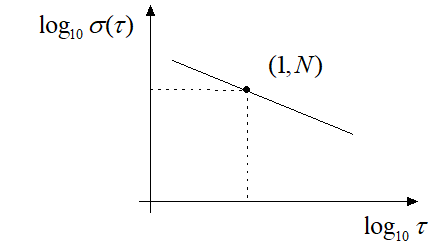角度随机游走是陀螺仪非常重要的一个噪声参数，在卡尔曼滤波中设置P阵中需要辨识这一参数。如果使用的是陀螺仪角增量信号，因为增量输出是一个积分过程，所以Allan方差的角度随机游走系数和采样频率无关，但是如果直接采样角速率数据，建议尽量提高采样频率来减少信息的损失，比如取两倍的带宽频率，能达到4到6倍或者以上更佳。
3．零偏不稳定性噪声（Bias Instability，BI）
根据《光纤陀螺仪指标（国军标）》的定义，零偏不稳定性主要表征了光纤陀螺输出量围绕其均值变化的离散程度。其功率谱密度与频率成反比，零偏不稳定性噪声的角速率功率谱为：
$S_{\Omega}(f)=B^{2} /(2 \pi f)$
上式中$B$为零偏不稳定性系数，对应的方差计算公式如下：
$\sigma_{B I}^{2}(\tau)=4 \int_{0}^{\infty} \frac{B^{2}}{2 \pi f} \frac{\sin ^{4}(\pi f \tau)}{(\pi f \tau)^{2}} \mathrm{d} f=\frac{2 B^{2}}{\pi} \int_{0}^{\infty} \frac{\sin ^{4}(\pi f \tau)}{(\pi f \tau)^{3}} \mathrm{d}(\pi f \tau) \approx \frac{4 B^{2}}{9}$
所以，在$\tau-\sigma_{B I}(\tau)$双对数图上，零偏不稳定性的斜率为0，它（或延长线）与 $\tau=1$的交点纵坐标读数为$2 B / 3$，如下图所示。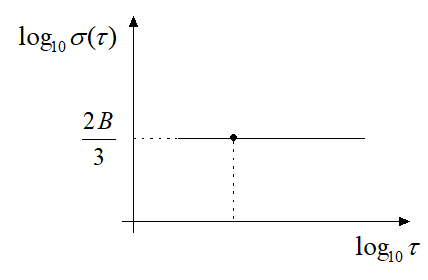零偏不稳定性噪声具有低频特性，在陀螺输出中表现为零偏随时间的缓慢波动。
4．角速率随机游走（Rate Random Walk，RRW）
和角速度随机游走的概念类似，如果陀螺角加速率建模为高斯白噪声，则角速率表现为随机游走现象。
角加速率的功率谱为：
$S_{\Omega^{\prime}}(f)=K^{\prime}$
根据功率谱相关性质，得到角速率的功率谱公式如下：
$S_{\Omega^{\prime}}(f)=K^{\prime}$
所以：
$\sigma_{R R W}^{2}(\tau)=4 \int_{0}^{\infty} \frac{K^{2}}{(2 \pi f)^{2}} \cdot \frac{\sin ^{4}(\pi f \tau)}{(\pi f \tau)^{2}} \mathrm{d} f=\frac{K^{2} \tau}{\pi} \int_{0}^{\infty} \frac{\sin ^{4}(\pi f \tau)}{(\pi f \tau)^{4}} \mathrm{d}(\pi f \tau)=\frac{K^{2} \tau}{3}$
因此，在$\tau-\sigma_{R R W}(\tau)$双对数图上，角速率随机游走的斜率为$1 / 2$，它（或延长线）与 $\tau=1$交点的纵坐标读数为$K / \sqrt{3}$，如下图所示。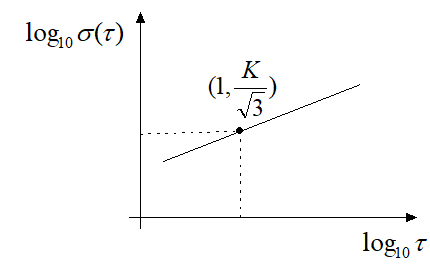5．速率斜坡（Rate Ramp，RR）
如果陀螺仪的角速率输出随时间缓慢变化，比如由于环境温度变化，角速率$\Omega$与测试时间$t$之间呈现线性关系，即：
$\Omega(t)=\Omega(0)+R t$
上公式中$R$为速率斜坡系数，根据艾伦方差的定义公式：
$y_{i}(\tau)=\frac{\left[\Omega(0)+R t_{i}\right]+\left[\Omega(0)+R\left(t_{i}+\tau\right)\right]}{2}=\Omega(0)+R t_{i}+\frac{R \tau}{2}$
$y_{i+1}(\tau)=\frac{\left[\Omega(0)+R\left(t_{i}+\tau\right)\right]+\left[\Omega(0)+R\left(t_{i}+2 \tau\right)\right]}{2}=\Omega(0)+R t_{i}+\frac{3 R \tau}{2}$
所以有：$\sigma_{R R}^{2}(\tau)=\frac{1}{2}\left\langle\left[y_{i+1}(\tau)-y_{i}(\tau)\right]^{2}\right\rangle=\frac{1}{2}\left\langle(R \tau)^{2}\right\rangle=\frac{R^{2} \tau^{2}}{2}$
可见，当角速率误差随时间线性变化时，将在$\tau-\sigma_{R R}(\tau)$双对数图上得到斜率为1的直线，它（或延长线）与$\tau=1$的交点纵坐标读数为$\mathrm{R} / \sqrt{2}$，如下图所示。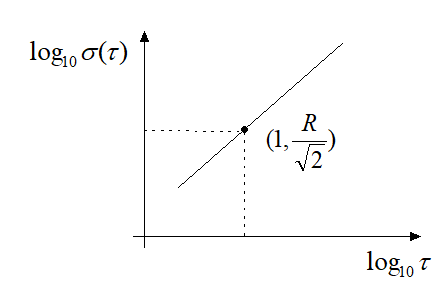实际上，角速率斜坡更像是一种确定性的误差，而不是随机误差。角速率斜坡常常由系统误差引起的，比如环境温度的缓慢变化，通过严格的环境温度控制或者引入补偿机制可以降低此类误差。
5种艾伦方差汇总介绍与单位转换
上面介绍了5种噪声参数和Allan方差之间的关系，实际系统输出信号的功率谱不一定是单一斜率的，而可能由几种统计独立的线性功率谱叠加组合而成，若将该总功率谱作为Allan方差滤波器的输入，则陀螺误差的Allan方差分析结果可写为：
$\sigma_{A}^{2}(\tau)=\sigma_{Q N}^{2}(\tau)+\sigma_{A R W}^{2}(\tau)+\sigma_{B I}^{2}(\tau)+\sigma_{R R W}^{2}(\tau)+\sigma_{R R}^{2}(\tau)$
整理可得到：
$\sigma_{A}^{2}(\tau)=\sigma_{Q N}^{2}(\tau)+\sigma_{A R W}^{2}(\tau)+\sigma_{B I}^{2}(\tau)+\sigma_{R R W}^{2}(\tau)+\sigma_{R R}^{2}(\tau)$
需要注意的是上公式是在国际单位制上推导出来的，上式中的 $\sigma_{A}(\tau)$和$\tau$的单位分为是 $r a d / s$和$s$，由此可得到各种噪声系数的单位，即：
$Q-\mathrm{rad}, \quad N-\mathrm{rad} / \mathrm{s}^{1 / 2}, \quad B-\mathrm{rad} / \mathrm{s}, \quad K-\mathrm{rad} / \mathrm{s}^{3 / 2}, \quad R-\mathrm{rad} / \mathrm{s}^{2}$
但是我们习惯上$\sigma_{A}(\tau)$常以$\left(^{\circ}\right) / \mathrm{h}$为单位，并且并且各项误差系数也常使用$°$和$h$的组合作为单位，为了达到该目的，根据换算关系$\operatorname{lrad} / \mathrm{s}=\frac{180 / \pi}{1 / 3600}\left(^{\circ}\right) / \mathrm{h}$} ，进行如下转换：
$\begin{array}{l} \sigma_{A}^{2}(\tau)\left(\frac{180 / \pi}{1 / 3600}\right)^{2}=\frac{3(Q \times 180 / \pi \times 3600)^{2}}{\tau^{2}}+\frac{\left[N \frac{180 / \pi}{(1 / 3600)^{1 / 2}} \times 60\right]^{2}}{\tau} \\ +\frac{4\left(B \frac{180 / \pi}{1 / 3600}\right)^{2}}{9}+\frac{\left[K \frac{180 / \pi}{(1 / 3600)^{3 / 2}} \times \frac{1}{60}\right]^{2} \tau}{3}+\frac{\left[R \frac{180 / \pi}{(1 / 3600)^{2}} \times \frac{1}{3600}\right]^{2} \tau^{2}}{2} \end{array}$
通过相应的变量替换：
\begin{aligned} &\sigma_{A}^{\prime}(\tau)=\sigma_{A}(\tau) \frac{180 / \pi}{1 / 3600}\left(\left(^{\circ}\right) / \mathrm{h}\right), Q^{\prime}=Q \times 180 / \pi \times 3600(")\\ &N^{\prime}=N \frac{180 / \pi}{(1 / 3600)^{1 / 2}}\left(\left(^{\circ}\right) / \mathrm{h}^{1 / 2}\right), \quad B^{\prime}=B \frac{180 / \pi}{1 / 3600}\\ &K^{\prime}=K \frac{180 / \pi}{(1 / 3600)^{3 / 2}}\left(\left(^{\circ}\right) / \mathrm{h}^{3 / 2}\right) \quad R^{\prime}=R \frac{180 / \pi}{(1 / 3600)^{2}}\left(\left({ }^{\circ}\right) / \mathrm{h}^{2}\right) \end{aligned}
整理可得：
$\sigma_{A}^{\prime 2}(\tau)=\frac{3 Q^{\prime 2}}{\tau^{2}}+\frac{\left(60 N^{\prime}\right)^{2}}{\tau}+\frac{4 B^{\prime 2}}{9}+\frac{\left(K^{\prime} / 60\right)^{2} \tau}{3}+\frac{\left(R^{\prime} / 3600\right)^{2} \tau^{2}}{2}=\sum_{k=-2}^{2} A_{k}^{\prime} \tau^{k}$
上式非常重要，有了它，当我们画出Allan方差曲线后，就可以直接通过读图的方式，辨识出各种噪声参数。
艾伦方差辨识参数的误差问题
理论上，Allan方差是随机过程一个现实的一种时间平均，但是由于实际应用时总是基于有限长度样本数据计算的，因而与经典方差估计一样，Allan方差也可看作是一种估计或统计量，服从一定的概率分布。研究表明，Allan方差估计$\hat{\sigma}_{A}(\tau)$的$1 \sigma$均方根误差可近似为（以相对百分比表示）
$E_{A}(\tau)=\frac{\left|\hat{\sigma}_{A}(\tau)-\sigma_{A}(\tau)\right|}{\sigma_{A}(\tau)} \approx \frac{1}{\sqrt{2(N / M-1)}} \times 100 \%$
上式中 $M$为样本长度，$N$为分组数据长度，$N / M$为分组数，上式表明表明分组数越少Allan方差的估计误差越大。
艾伦方差曲线的绘制方法
假设我们获得了一组平均角速率样本序列：
$\bar{\Omega}_{1}\left(\tau_{0}\right), \bar{\Omega}_{2}\left(\tau_{0}\right), \bar{\Omega}_{3}\left(\tau_{0}\right), \cdots, \bar{\Omega}_{N}\left(\tau_{0}\right)$
公式中$\tau_{0}$为采样间隔，一般IMU的采样频率一般在几百$Hz$甚至上千$Hz$,一般在计算艾伦方差时会静止放置几个小时，但是由于样本序列的长度总是有限的，因此Allan方差计算只能给出理论值的一个估计。
下面给出实现Allan方差计算的具体步骤：

首先计算取样时间为$\tau_{0}$时的Allan方差：
$\hat{\sigma}_{A}^{2}\left(\tau_{0}\right)=\frac{1}{2(N-1)} \sum_{k=1}^{N-1}\left[\bar{\Omega}_{k+1}\left(\tau_{0}\right)-\bar{\Omega}_{k}\left(\tau_{0}\right)\right]^{2}$
其次将取样时间间隔加倍，记$\tau_{1}=2^{1} \tau_{0}$和$N_{1}=[N / 2]$，（$[\cdot]$表示取整），在相继奇偶序号角速率之间作算术平均，即
$\bar{\Omega}_{k}\left(\tau_{1}\right)=\frac{\bar{\Omega}_{2 k-1}\left(\tau_{0}\right)+\bar{\Omega}_{2 k}\left(\tau_{0}\right)}{2} \quad k=1,2,3, \cdots, N_{1}$
组成新的取样时间间隔为$\tau_{1}$的平均角速率序列，即：
$\bar{\Omega}_{1}\left(\tau_{1}\right), \bar{\Omega}_{2}\left(\tau_{1}\right), \bar{\Omega}_{3}\left(\tau_{1}\right), \cdots, \bar{\Omega}_{N_{1}}\left(\tau_{1}\right)$
显然新序列的长度减半（但可能相差1个数据，下同），计算取样时间为$\tau_{1}$时的Allan方差，即：
$\hat{\sigma}_{A}^{2}\left(\tau_{1}\right)=\frac{1}{2\left(N_{1}-1\right)} \sum_{k=1}^{N_{1}-1}\left[\bar{\Omega}_{k+1}\left(\tau_{1}\right)-\bar{\Omega}_{k}\left(\tau_{1}\right)\right]^{2}$
再次将取样时间间隔加倍，记$\tau_{2}=2 \tau_{1}=2^{2} \tau_{0}$和$N_{2}=\left[N_{1} / 2\right]$ ,计算平均角速率，即：
$\bar{\Omega}_{k}\left(\tau_{2}\right)=\frac{\bar{\Omega}_{2 k-1}\left(\tau_{1}\right)+\bar{\Omega}_{2 k}\left(\tau_{1}\right)}{2} \quad k=1,2,3, \cdots, N_{2}$
组成新的取样时间间隔为$\tau_{2}$的平均角速率序列，即：
$\bar{\Omega}_{1}\left(\tau_{2}\right), \bar{\Omega}_{2}\left(\tau_{2}\right), \bar{\Omega}_{3}\left(\tau_{2}\right), \cdots, \bar{\Omega}_{N_{2}}\left(\tau_{2}\right)$
新序列的长度再次减半，计算取样时间为KaTeX parse error: Can't use function '$' in math mode at position 9: \tau_{2}$̲，时的Allan方差，即：
\hat{\sigma}{A}^{2}\left(\tau{2}\right)=\frac{1}{2\left(N_{2}-1\right)} \sum_{k=1}{N_{2}-1}\left[\bar{\Omega}_{k+1}\left(\tau_{2}\right)-\bar{\Omega}_{k}\left(\tau_{2}\right)\right]{2}
如此反复将取样时间间隔不断加倍，记$\tau_{L}=2 \tau_{L-1}=2^{L} \tau_{0}$和$N_{L}=\left[N_{L-1} / 2\right]$,直至最终序列的长度不小于2，得平均角速率序列为:
$\bar{\Omega}_{1}\left(\tau_{L}\right), \cdots, \bar{\Omega}_{N_{L}}\left(\tau_{L}\right)$
并计算取样时间为$\tau_{L}$时的Allan方差，即:
$\hat{\sigma}_{A}^{2}\left(\tau_{L}\right)=\frac{1}{2\left(N_{L}-1\right)} \sum_{k=1}^{N_{L}-1}\left[\bar{\Omega}_{k-1}\left(\tau_{L}\right)-\bar{\Omega}_{k}\left(\tau_{L}\right)\right]^{2}$
至此获得一系列的点对，$\tau_{i} \sim \hat{\sigma}_{A}^{2}\left(\tau_{i}\right)$或$\tau_{i} \sim \hat{\sigma}_{A}\left(\tau_{i}\right)$，$(i=1,2,3, \cdots, L)$，完成Allan方差估计，并将结果绘制成双对数图。
从上述计算过程可以看出，在$\tau_{0}$基础上取样间隔时间是以2的倍数递增的，即取样时间为2的幂次方倍，而在一般应用中不需计算其他时间间隔上的Allan方差值。还容易看出，Allan方差的计算过程比较简单，并且计算量也不大。

艾伦方差相关代码
下面给出艾伦方差曲线绘制的matlab代码，可以对应上面的步骤。
function [sigma, tau, Err] = avar(y0, tau0)
% 计算Allan方差
% 输入：y -- 数据(一行或列向量), tau0 -- 采样周期
% 输出：sigma -- Allan方差(量纲单位与输入y保持一致), tau -- 取样时间, Err -- 百分比估计误差
% 作者: Yan Gong-min, 2012-08-22
% example:
%     y = randn(100000,1) + 0.00001*[1:100000]';
%     [sigma, tau, Err] = avar(y, 0.1);
N = length(y0);
y = y0; NL = N;
for k = 1:inf
sigma(k,1) = sqrt(1/(2*(NL-1))*sum([y(2:NL)-y(1:NL-1)].^2));
tau(k,1) = 2^(k-1)*tau0;
Err(k,1) = 1/sqrt(2*(NL-1));
NL = floor(NL/2);
if NL<3
break;
end
y = 1/2*(y(1:2:2*NL) + y(2:2:2*NL));  % 分组长度加倍(数据长度减半)
end
subplot(211), plot(tau0*[1:N], y0); grid
xlabel('\itt \rm/ s'); ylabel('\ity');
subplot(212),
loglog(tau, sigma, '-+', tau, [sigma.*(1+Err),sigma.*(1-Err)], 'r--'); grid
xlabel('\itt \rm/ s'); ylabel('\it\sigma_A\rm( \tau )');

从艾伦方差曲线中辨识噪声参数可以通过手动读图的方式，当然也可以通过自动化的形式，自动辨识出参数，下面代码是一个自动辨识角度随机游走参数的代码，主要步骤是：通过辨识的噪声参数对应到log曲线频率，通过查找最为接近的频率对应的纵坐标数据。
需要注意的是下面代码输入的陀螺仪单位是国际单位，为$r a d / s$，如果使用$\operatorname{deg} / h$，需要注意单位的转换。
%% Noise Parameter Identification
% Find the index where the slope of the log-scaled Allan deviation is equal to the slope specified.

% Angle Random Walk
% The above equation is a line with a slope of -1/2 when plotted on a log-log plot of σ(τ)versusτ.
% The value of N can be read directly off of this line at τ=1.
slope = -0.5;
logtau = log10(tau);
[~, i] = min(abs(dlogadev - slope)); %找到slope=-0.5 最接近的点；

% Find the y-intercept of the line.
% Determine the angle random walk coefficient from the line.
logN = slope*log(1) + b;
% disp('random walks');

% Plot the results.
tauN = 1;
lineN = N ./ sqrt(tau);
figure
loglog(tau, adev, tau, lineN, '--', tauN, N, 'o')
title('Allan Deviation with Angle Random Walk')
xlabel('\tau')
ylabel('\sigma(\tau)')
legend('\sigma', '\sigma_N')
text(tauN, N, 'N')
grid on
axis equal

艾伦方差应用实例

In-Run Bias Stability: $2.5^{\circ} / h$
Angular Random Walk: $0.15^{\circ} / \sqrt{h}$
另外还可以看到其零偏重复性是$0.4^{\circ} / \mathrm{sec}$，，还是挺大的，所以一般需要在开始上电后静置数秒得到零偏重复性。根据国军标的定义，零偏重复性为：在同样条件下及规定间隔时间内，多次通电过程中，陀螺仪零偏相对其均值的离散程度，以多次测试所得零偏的标准偏差表示。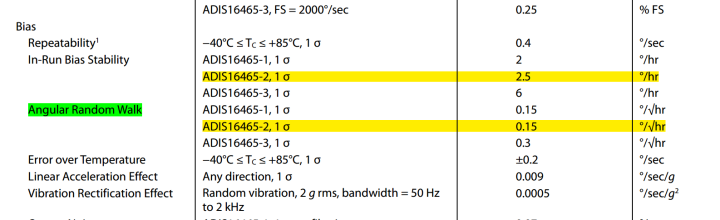下图是ADIS16465静置2.5小时$Z$轴的数据，采样率为$200Hz$，从图中可以看出：曲线大致可以分成三段，最左边的斜率为 $-1/2$，即角度随机游走噪声，因为这里的纵坐标单位是$°/h$，横坐标$\tau=1$对应的纵坐标为 $7.2^{\circ} / h$，根据前面介绍的如下公式：
$\sigma_{A}^{\prime 2}(\tau)=\frac{3 Q^{\prime 2}}{\tau^{2}}+\frac{\left(60 N^{\prime}\right)^{2}}{\tau}+\frac{4 B^{\prime 2}}{9}+\frac{\left(K^{\prime} / 60\right)^{2} \tau}{3}+\frac{\left(R^{\prime} / 3600\right)^{2} \tau^{2}}{2}=\sum_{k=-2}^{2} A_{k}^{\prime} \tau^{k}$
从上式可知：
$N=\sigma_{A R W} \cdot \frac{\sqrt{\tau}}{60}=\sigma_{A R W} \sqrt{\frac{1}{3600} h}=\frac{\frac{7.2^{\circ}}{\sqrt{h}}}{60}=\frac{0.12^{\circ}}{\sqrt{h}}$
所以和数据手册中的Angular Random Walk相差不大。
下面看零偏不稳定性，斜率为0对应的横坐标为 $41s$，纵坐标为$2.4°/h$ ，所以
$B=2.4^{\circ} /(2 / 3) / h=3.6^{\circ} / h$
可以看到和数据手册也相差不大，因为艾伦方差只能当成一种粗略的参数辨识手段，所以为了方便识图，这里当成$2.4°/h$ 更简单点。
下面看角速率随机游走参数，这里看横坐标为$100s$时的数据，对应的纵坐标为$3.0°/h$，所以：
$K=\frac{60 \sqrt{3} \sigma_{R R W}}{\sqrt{\tau}}=\frac{60 \sqrt{3} \cdot 3.0}{\sqrt{100}} / h^{3 / 2}=31^{\circ} / h^{3 / 2}$
另外图中显示的数据平均值为$329°/h$，因为16465的零偏稳定性很小，只有$3.0°/h$，基本可以忽略不计，所以零偏重复性大概为$0.09^{\circ} / \mathrm{sec}$，小于数据手册中的$0.4^{\circ} / \mathrm{sec}$，但是仍然说明零偏重复性对系统影响巨大，在实际应用中需要很好的处理。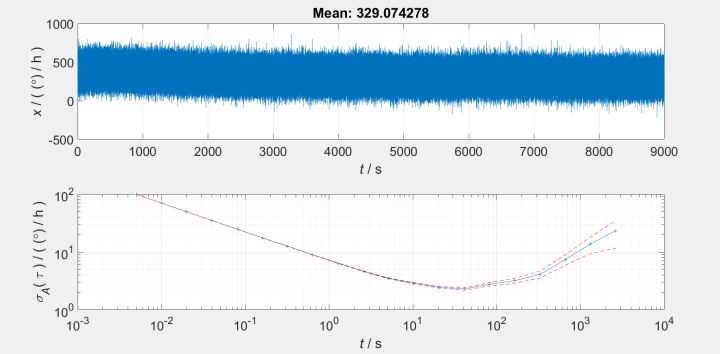下面是加速度三个轴的艾伦方差曲线，纵坐标单位为$g$，从图中可以看出，横坐标0.1到10之间的曲线的斜率大约为$-1/2$，所以该段曲线为速度随机游走误差， 横坐标$10^{0}$所对应的的纵坐标大约为$20 u g$，即$0.012 \frac{m}{s \sqrt{h}}$， 和数据手册给出的值相当。
横坐标为10 的地方的斜率大约为0，所以该点对应的是零偏不稳定性噪声，读图可知，零偏不稳定性噪声 X轴略大，为$34 u g$，Y轴和Z轴轴的数据大致相同，为$20 u g$，比数据手册中给出的略大。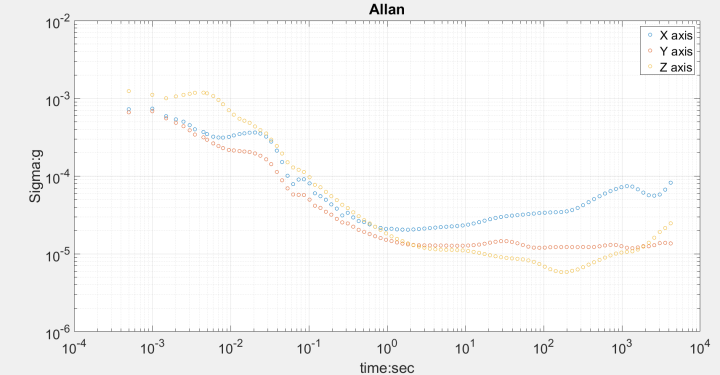下图是ADIS16470静置2小时Z轴的采样的数据，采样率为$200 H z$，可以看到大致可以分成两段，角速率随机游走参数已经很小了。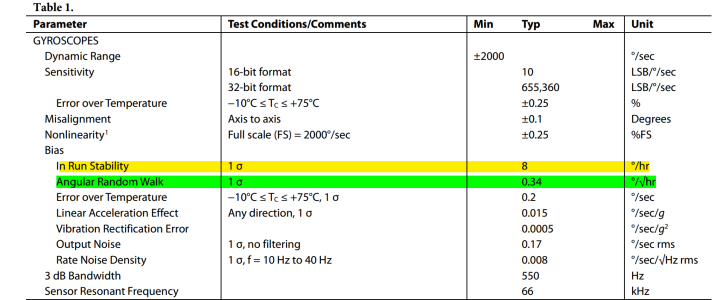数据手册参数如下：

In-Run Bias Stability:$8^{\circ} / h$
In-Run Bias Stability:$0.34^{\circ} / \sqrt{h}$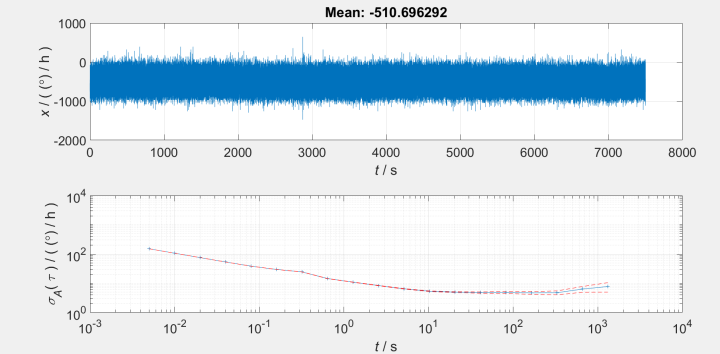$\begin{array}{c} N=\sigma_{A R W} \cdot \frac{\sqrt{\tau}}{60}=\sigma_{A R W} \sqrt{\frac{1}{3600} h}=\frac{\frac{12^{\circ}}{\sqrt{h}}}{60}=\frac{0.2^{\circ}}{\sqrt{h}} \\ B=5^{\circ} /(2 / 3) / h=7.5^{\circ} / h \end{array}$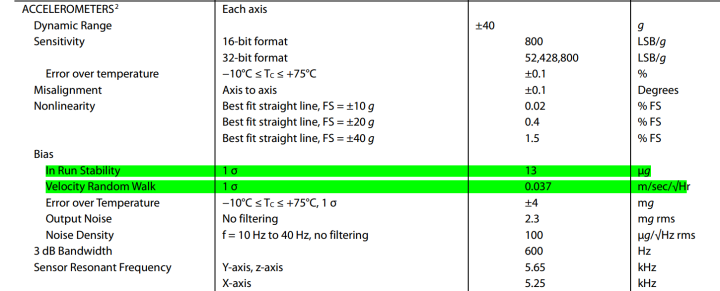下图是ADIS16470的加速度艾伦方差曲线，可以看到横坐标10之前的曲线的斜率大概为 $-1 / 2$，即为速度随机游走部分曲线，当横坐标为1时，纵坐标为$60 u g$，可得速度随机游走噪声为：$0.036 \frac{m}{s \sqrt{h}}$，和数据手册中的 噪声相当。
当横坐标为10时，斜率为0，对应的是零偏不稳定性，可得零偏不稳定性噪声大约为$45 u g$，比数据手册中的参数略大。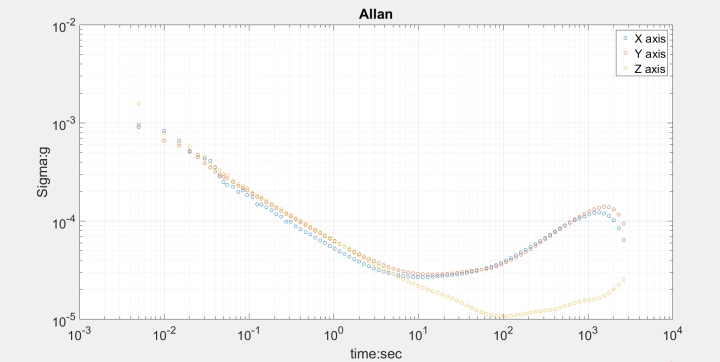3.某型号光纤惯导
下图是光纤陀螺静置1.5个小时的采样数据，采样率为$100 H z$，可以看出曲线的后半段为角度随机游走噪声，零偏不稳定性在图中没有显示出来，可能采样时间还是短了些。
$N=\sigma_{A R W} \cdot \frac{\sqrt{\tau}}{60}=0.14 \cdot \frac{\sqrt{100^{\circ}}}{60 \sqrt{h}}=\frac{0.0023^{\circ}}{\sqrt{h}}$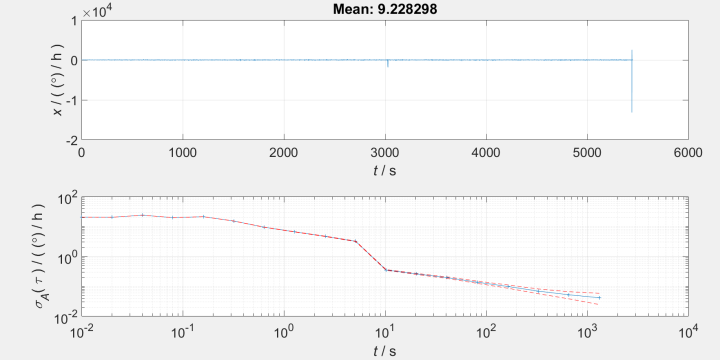另外从光纤陀螺的的数据均值可以看出，相比Mems陀螺仪的零偏重复性已经非常小了，图中的均值并不能反映零偏，因为没有去除地球自转角速度在Z轴的分量。
10s平滑计算零偏不稳定性
国军标中常用10s平滑方法计算零偏不稳定性，这里比较下它和艾伦方差在计算零偏稳定性之间的数值差异。10s平滑主要思路是每10s计算下均值，再计算这些均值的方差，例如有1000s的数据，需要计算100个均值，并求这100个均值的方差。主要参考代码如下：
for i=1:3
%Bias stability and Bias
Smo=10;%N 10秒平滑
num=Smo/t0;%%m每组内数据个数
m=floor(length(Wb(:,i))/num);%%共可以分成m组数据
gx=zeros(m,1);
Xbais_all = mean(Wb(:,i));
for j=1:m-1
gx(j)=mean(Wb(1+(j-1)*num:1+j*num,i)); %取平均
end
gx= gx(2:end-2,:);
Xbais_stability = std(gx); % 计算标准差
fprintf('Bias Stability 10s:%0.2e [deg/h]\n',Xbais_stability);
end


光纤陀螺的10s平滑结果为：$4.62^{\circ} / h$
ADIS16470的10s平滑结果为：$13.3^{\circ} / h$；艾伦方差：$7.5^{\circ} / h$
ADIS16465的10s平滑结果为：$29.1^{\circ} / h$；艾伦方差：$3.6^{\circ} / h$

可看到10平滑方法计算出来的零偏稳定性相对艾伦方差较大，不知道有没有理论证明。
下面是加速度计的10s平滑结果：

ADIS16465：$X$轴$99 u g$，$Y$轴$26u g$，$Z$轴$29 u g$;
ADIS16470：$X$轴$4.24 m g$，$Y$轴$6.17m g$，$Z$轴$0.46 m g$;
光纤惯导：$X$轴$97 u g$，$Y$轴$53u g$，$Z$轴$150 u g$;

参考文献

惯性仪器测试与数据分析 严恭敏
Analysis and Modeling of Inertial Sensors Using Allan Variance


展开全文惯性导航
• 8.11 基于粒子群算法的机械手参数辨识 本节介绍采用粒子群算法实现8.8节中机械手的参数辨识。由式(8.22)可知 (8.29) 其中Y 和 的表达式见式(8.20)。 需要辨识的参数向量为 ，其真实...
• Python写的 在网上找的代码，改了一下，权重随训练变化的 改的有点乱，请多担待 训练参数误差能到2.89%
• 码下此文章，只因在各大搜索都难以找到多变量最小二乘参数辨识MATLAB代码 然后！希望我的课程作业可以帮助到还在苦苦寻找多变量最小二乘参数辨识MATLAB代码的你 1. 多重最小二乘参数辨识(RLS) （理论有空更…一定...matlab
• 系统参数辨识常用的是最小二乘法，但最小二乘法存在限制需要改进，该包中含常用的方法如递推最小二乘法，遗忘因子最小二乘法，增广最小二乘法，辅助变量法的matlab代码
• 工业机器人的实时非线性参数识别。 代码包括用于单批次优化的度量和算法。 该贡献已提交给IROS2021。该代码具有三个入口点： MASTER_identification 根据记录的运动开始识别参数。 MASTER_create_symbolic_robot ...C
• RLS递归最小二乘，在线辨识参数，包括相关文档和代码，适合新手学习，代码可直接在matlab上运行。
• 本书从MATLAB仿真角度系统介绍了系统辨识的基本理论、基本方法和应用技术，系统辨识常用输入信号、最小二乘参数辨识方法及原理、极大似然参数辨识方法及其应用、传递函数的时域和频域辨识、神经网络辨识及其应用、...
• ## 系统辨识的几种方法实现MATLAB代码

千次阅读 多人点赞 2019-01-28 17:06:10
系统辨识，即在已知系统阶次的情况下辨识出系统的参数辨识系统阶次，一般用到的方法有最小二乘法、辅助变量法等，对于离线与在线辨识，只是加入一个递推的问题。下面本文给出系统辨识方法的实现代码。 首先是处理...MATLAB
• 代码是我的毕设里面的一部分...对其中的惯性参数进行辨识，将模型的惯性参数分为六个未知量，在已知位置，速度，加速度和力矩的情况下，进行递推就可以最终得到辨识的六个参数。 有关递推最小二乘的原理，可以参考刘matlab
• 代码写的很不错，正需要呢 系统参数辨识 ARMAX
• 用MATLAB来做最小二乘参数辨识，含有代码，结果分析。
• 作业一 递推最小二乘法参数辨识 设被辨识系统的数学模型由下式描述： 式中x(k)为方差为0.1的白噪声。要求： 当输入信号u(k)是方差为1的白噪声序列时，利用系统的输入输出数据在线辨识上述模型的参数； ...
• 资源为优化算法差分进化算法的一个代码，与系统辨识，可以辨识参数，效果不错
• 基于matlabGUI下面的飞机参数识别，包括气动参数识别，提供demo和相关说明，F16的相关代码，图形化界面输入
• LR手动关联参数化问题总结《转载》所谓的关联就是把脚本中某些写死的代码(hard-coded)数据，转变成截取自服务器所送的、动态的、每次都不一样的数据。一般情况下，比较聪明的服务器在每个浏览器第一次跟它要数据时，...
• LR手动关联参数化问题总结所谓的关联就是把脚本中某些写死的代码(hard-coded)数据，转变成截取自服务器所送的、动态的、每次都不一样的数据。一般情况下，比较聪明的服务器在每个浏览器第一次跟它要数据时，都会在...
• 用一步完成最小二乘法、递推最小二乘法、增广最小二乘法、广义最小二乘法、辅助变量法、二步法辨识如下模型的参数： 噪声的成形滤波器 采样时间0.01 要求： 1.用matlab 写出程序代码； 2.画出实际模型和...最小二乘法 递推最小二乘法 增广最小二乘法
• indent可辨识C的原始代码文件，并加以格式化，以方便程序设计师阅读。 indent命令按照随命令输入的标志所指定的格式重新格式化一个 C 程序。 语法格式：indent [参数] 常用参数： -bad 在声明区段或加上空白行 ...
• 语音识别MFCC特征提取matlab代码。...「梅尔倒频谱系数」（Mel-scale Frequency Cepstral Coefficients，简称MFCC），是最常用到的语音特征，此参数考虑到人耳对不同频率的感受程度，因此特别适合用在语音辨识
• Matlab代码写成τ=Yp的形式(τ-关节扭矩 p-参数集) % 三连杆机械臂瞬态运动的牛顿-欧拉递归逆动力学求解： % 参数：（运动指令）各关节运动角度， 关节速度， 关节加速度（3*1矩阵） % 返回值：各关节力矩（3*1...matlab 数学建模